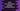# How to use fold, foldIndexed, foldRight and foldRightIndexed in Kotlin# How to use fold, foldIndexed, foldRight and foldRightIndexed in Kotlin :

If you want to do certain operation on the elements of a list serially, you can use fold(). This method takes one initial value and one operation to perform on the elements serially starting from the provided initial value. fold() performs the operation from left to right. If you want to do it from right to left, you can use foldRight().

Similarly, if we want to get access of the current index while iterating, we can use use foldIndexed and foldRightIndexed respectively. In this tutorial, we will learn how to use fold, foldIndexed, foldRight and foldRightIndexed in Kotlin with example.

## fold() :

Example :

``````fun main(args: Array) {
val total = listOf(1, 2, 3, 4, 5).fold(0) { currentValue, result -> result + currentValue }
print(total)
}``````

It will print 15 as the output.

As you can see, here 0 is the initial value and we are adding all values of the list starting 0. Now, if we change the above program like below :

``````fun main(args: Array) {
val total = listOf(1, 2, 3, 4, 5).fold(10) { currentValue, result -> result + currentValue }
print(total)
}``````

It will print 25 as the output. Because, we are using 10 as starting value in this example.

## foldRight() :

foldRight() is same as fold(). The only difference is that it will iterate from right to left instead of left to right as fold(). The above example will produce the same result for foldRight(). To find out that it moves from right to left, let me show you with a different example :

``````fun main(args: Array) {
val s = listOf(100,5,7,8).foldRight(10) { currentValue, _ -> currentValue }
print(s)
}``````

It will print 100 as the output. Because we are setting the current value as the final value on each step. Since the leftmost value is 100, it will be the last as it moves from right to left.

## foldIndexed() :

You can see that we don’t have access to the current index in the examples above. If you want to know the current index while iterating, you can use foldIndexed() instead of fold() (for left to right iteration).

Similarly, we can use foldRightIndexed method to fold from right to left.

### Conclusion :

fold() is a useful method to quickly do any operation on all elements of a list or map. This method comes in handy if you don’t want to use loop for iterating the elements. Try to run the examples we have shown above and drop one comment below if you have any queries.

You might also like :